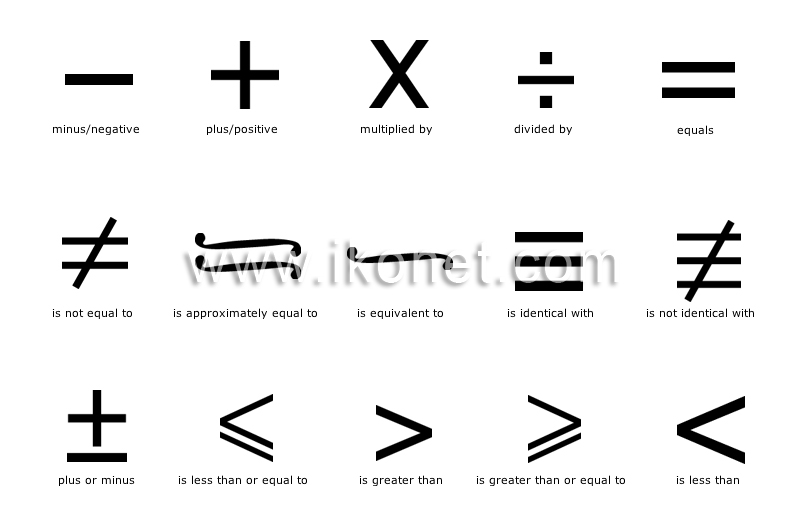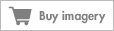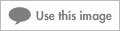Look up an image:

#### mathematicsThe science that uses deductive reasoning to study the properties of abstract entities such as numbers, space and functions and the relations between them.See mathematics in : french | spanish

#### is greater thanSign denoting that the value on the left is of greater magnitude than the number that follows.

#### is greater than or equal toSign denoting that the result of an operation is equal to or of greater magnitude than the number that follows.

#### is less thanSign denoting that the value on the left is of smaller magnitude than the number that follows.

#### is less than or equal toSign denoting that the result of an operation is equal to or of smaller magnitude than the number that follows.

#### plus or minusSign denoting that the number that follows denotes an order of magnitude.

#### is identical withBinary sign denoting that the result of the operation noted on the left has the same value as the operation noted on the right.

#### is not identical withBinary sign denoting that the result of the operation noted on the left does not have the same value as the operation noted on the right.

#### equalsSign denoting the result of an operation.

#### is not equal toSign denoting that the result of an operation is not close to the same value as the one on the right.

#### is approximately equal toSign denoting that the result of an operation is close to the same value as the one on the right.

#### is equivalent toSign denoting that the value on the left is the same magnitude as the one on the right.

#### divided bySign denoting a number (dividend) is to be divided by another (divisor); the result is a quotient.

#### multiplied bySign denoting that a number is to be multiplied by another; the result is a product.

#### minus/negativeSign denoting that a number is to be subtracted from another; the result is a difference.

#### plus/positiveSign denoting that a number is to be added to another; the result is a sum.